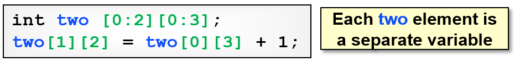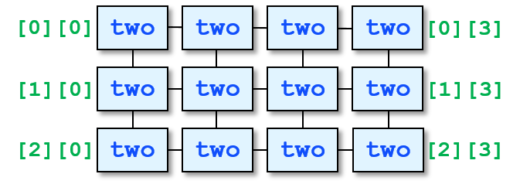# SystemVerilog Multidimensional Arrays

### You asked and I listened

Thank you everyone who registered and attended my webinar on SystemVerilog arrays. There were many great questions and I’ve answered many of them here. “SystemVerilog arrays” is a big topic and I had to leave out many ideas. There were several questions on Multidimensional Arrays (MDAs), so here is a very short introduction. Copy and paste this code and run on your favorite simulator. Get dirty, make mistakes, debug – you are a verification engineer so figure it out!

### Exploring the next dimension

Let’s start with a one dimensional array, fixed size, with 4 elements and some code to initialize it.

```int one;
foreach (one[i]) one[i] = i;```

The best way to think about MDAs is that they are arrays of arrays. So a two dimensional array like the following is made of 3 arrays, each which has 4 elements.

```int two; // Short declaration, equivalent to ...```

Here is its layout in memory.You can assign three copies of the one array to it.

```two = '{ 3 { one } };
\$display("two = %p", two); // '{'{0, 1, 2, 3}, '{0, 1, 2, 3}, '{0, 1, 2, 3}}
```

### Stepping through MDAs

By now you know that my favorite way to step through an array is with a foreach loop. SystemVerilog has a quirk here – the foreach has a comma separated list of index variables, not separate bracketed indexes. Here is an example.

```foreach (two[i,j]) // Not two[i][j]
\$display("two[%0d][%0d]=%0d", i, j, two[i][j]);```

Here is the output. You can see that the right-most dimension varies the fastest.

```two=0
two=1
two=2
two=3
two=0
two=1
two=2
two=3
two=0
two=1
two=2
two=3```

### Hip to be (not) square

You can mix array types with MDAs. How about a fixed size array that contains several dynamic arrays? Better yet, the dynamic arrays don’t have to be the same size, so the final array could be triangular!

```int triangle[];
initial begin
\$display("Start: triangle = %p\n", triangle);

foreach (triangle[i]) begin
\$display("Construct: triangle[%0d]", i);
triangle[i] = new[i+1];
end
\$display("Constructed: triangle = %p\n", triangle);

foreach (triangle[i,j]) begin
\$display("Initialize: triangle[%0d][%0d]", i, j);
triangle[i][j] = i*10 + j;
end
\$display("Final: triangle = %p", triangle); // '{'{0}, '{0, 0}, '{0, 0, 0}}
end```

### Scoreboard with multiple matches

When you are building a testbench, your scoreboard needs to save the expected results until they are compared with the actual values from the design. If it can reorder transactions, you can store transactions in an associative array so you can easily look them up, based on a key value that won’t change as the transaction moves through the system. For example, there might be an address field, so store the transactions in an associative array indexed by the address.

That works well until two transactions have the same address, so they both need to be stored in the same location in the associative array, which is not possible. So instead, make every element a queue of all the transactions with that single address. I’ve been saying this for decades, but never actually did this. Turns out to be trivial! First, here is a simplified version with just integers.

```int sb[int][\$];
initial begin
\$display("Start: sb = %p\n", sb);
for (int i=0; i<22; i=i+2) begin
sb[i%10].push_front((i/10)*10);
end
\$display("Init: sb = %p\n", sb);
end```

Now here is a more elaborate example. The transaction class has address and data properties. If you construct an object with new(12), the constructor splits the value into the 10’s and the 1’s digits, so the data is 10 and the address is 2.

```typedef bit[23:0] addr_t;
class Xact;
int data;
function new(input int i);
addr = i%10;        // Use one's digit
data = (i/10) * 10; // Use 10's digit
endfunction
endclass```

Here is the scoreboard and a temporary handle, and a function to add an element.

```Xact scoreboard[addr_t][\$], t;

endfunction```

Finally, the following code fills the scoreboard with the transactions for the values 0, 1, 2, … 21.

```  initial begin
// Fill scoreboard with 0, 1, 2, ... 21
for (int i=0; i<22; i++) begin
t = new(i);
end```
```     // Display the scoreboard contents
foreach (scoreboard[i,j]) begin
if (j==0) \$write("\n scoreboard[%0d] = ", i);
\$write("%0d ", scoreboard[i][j].data);
end
end```

Try this out with your favorite simulator, especially if it starts with Q.